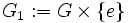# Normal not implies characteristic in the collection of all groups satisfying a nontrivial finite direct product-closed group property

## Statement

Suppose$\mathcal{V}$ is the subcollection of the collection of all groups that satisfies a finite direct product-closed group property and contains at least one nontrivial group. Then, there exists a group$K \in \mathcal{V}$ and a subgroup$G$ of$K$, such that$G$ is a Normal subgroup (?) of$K$ but not a Characteristic subgroup (?) of$K$.

Note that this in particular applies to the situation where$\mathcal{V}$ is a subvariety or a subquasivariety of the variety of groups.

## Particular cases

Normal not implies characteristic in:

• the collection of finite groups.
• the collection of abelian groups.
• the collection of finite$p$-groups for any prime number$p$.

and all bigger varieties/quasivarieties.

## Proof

### Example of a direct product

Let$G$ be any nontrivial group in$\mathcal{V}$. Then consider$K = G \times G$, viz., the external direct product of$G$ with itself. The subgroups$G_1 := G \times \{ e \}$ and$G_2 := \{ e \} \times G$ are direct factors of$K$, and are hence both normal in$K$. Note also that they are distinct, since$G$ is nontrivial.

However, the exchange automorphism:$(x,y) \mapsto (y,x)$

exchanges the subgroups$G_1$ and$G_2$. Thus, neither$G_1$ nor$G_2$ is invariant under all the automorphisms, so neither is characteristic. Thus,$G_1$ and$G_2$ are both normal subgroups of$K$ that are not characteristic.

Note that this example also shows that direct factor does not imply characteristic subgroup.

## GAP implementation

### Implementation of the generic example

Before using this generic example, you need to define$G$ for GAP, choosing any nontrivial group (double semicolons have been used here to suppress GAP's output for the first three commands, which depends on the specific choice of$G$ -- you can use single semicolons instead).

gap> K := DirectProduct(G,G);;
gap> G1 := Image(Embedding(K,1));;
gap> G2 := Image(Embedding(K,2));;
gap> IsSubgroup(K,G1);
true
gap> IsSubgroup(K,G2);
true
gap> IsNormal(K,G1);
true
gap> IsNormal(K,G2);
true
gap> IsCharacteristicSubgroup(K,G1);
false
gap> IsCharacteristicSubgroup(K,G2);
false